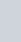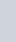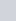The probability of guessing the correct answer to a certain test questions is
Question:

The probability of guessing the correct answer to a certain test questions is $\frac{x}{12}$. If the probability of not guessing the correct answer to this question is $\frac{2}{3}$, then $x=$

(a) 2

(b) 3

(c) 4

(d) 6

Solution:

GIVEN: Probability of guessing a correct answer to a certain question isProbability of not guessing a correct answer to a same questionTO FIND: The value of x

CALCULATIONWe know that sum of probability of occurrence of an event and probability of non occurrence of an event is 1.

If E is an event of occurrence andis its complementary then

$P(E)+P(\bar{E})=1$

According to the question we have

$\frac{x}{12}+\frac{2}{3}=1$

$\frac{x+8}{12}=1$

$x+8=12$

$x=4$

Hence the correct option is $(c)$